# Algebra II : Basic Statistics

## Example Questions

### Example Question #31 : Basic Statistics

Observe the following list of terms: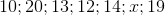The range of this list of numbers is. Which value cantake?Explanation:

The range of this list of numbers is 10 which is obtained by doing 20-10.

This means that 20 and 10 are respectively the maximum and minimum values of the list of numbers.

Therefore x has to be a value between 10 and 20.

17 being the only value within this range it is therefore the right answer.

### Example Question #32 : Basic Statistics

For a set of numbers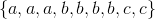where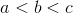and the set has the following values: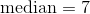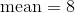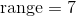Determine the value of.Explanation:

{6,6,6,7,7,7,7,13,13}

median=7 so b=7

mean=8 so the sum of the set is 72.

72-4(7)=44

44=3a+2c

Range=7  so c-a=7

44=3a+2(a+7)

a=6 and c=13.

### Example Question #31 : Basic Statistics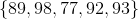Given the set of test scores, what is the range?Explanation:

The range is the difference between the smallest number and the largest number. The smallest value is 77, and the largest is 98.

98 – 77 = 21

### Example Question #32 : Basic Statistics

The range of the following data set is 18. What is a possible value for?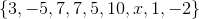Cannot be determined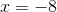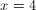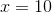Explanation:

Arrange the known values in the set in numerical order: {–5, –2, 1, 3, 5, 7, 7, 10}. The range is the difference between the largest value and smallest value.

x must be either the largest or the smallest value in the set.

range = x – smallest value

18 = x – (–5)

18 = x + 5

13 = x

OR

range = largest value – x

18 = 10 – x

8 = –x

–8 = x

### Example Question #33 : Basic Statistics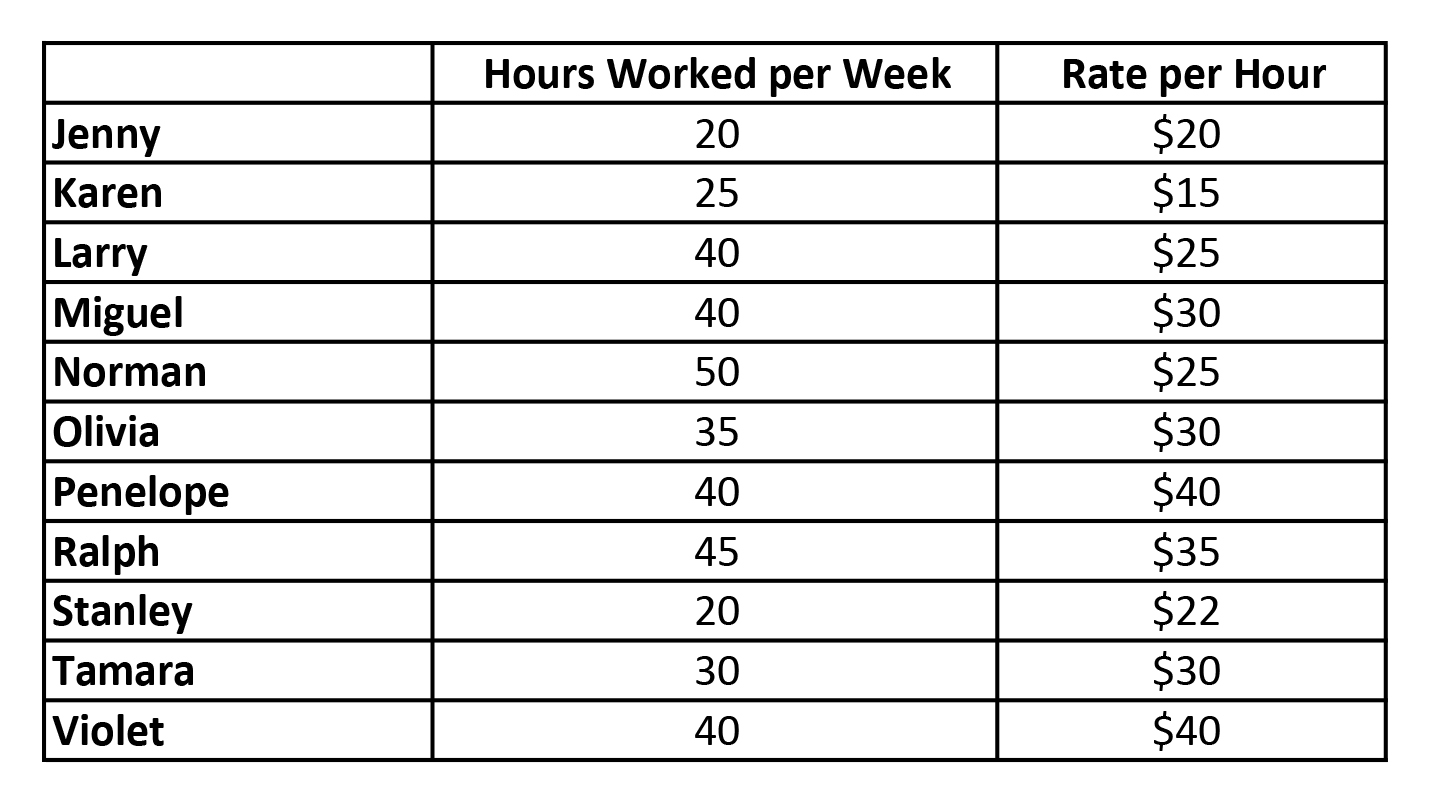The above chart shows a specific week of work at an advertising firm.  What is the range of the hourly rates of the workers?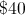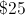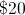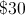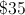Explanation:

The range is difference between the largest value and the smallest value.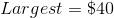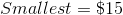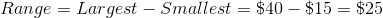### Example Question #1 : How To Find Range

What is the range of the set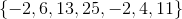?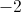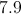Explanation:

The range is defined as the difference between the highest and lowest numbers in a set. Here, the highest number isand the lowest isTherefore, the range is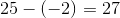is the mode,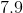is the mean, and 6 is the median.

### Example Question #2 : Range

Find the range of the set: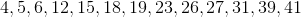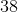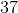Explanation:

To find the range of a set subtract the smallest number in the set from the largest number in the set: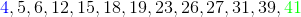The largest number is in green: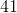The smallest number is in blue:Therefore the range is as follows.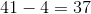### Example Question #1 : Data Properties

Find the range of the set: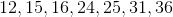Explanation:

To find the range of a set subtract the smallest number in the set from the largest number in the set: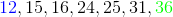The largest number is in green:The smallest number is in blue:Therefore the range is,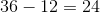.

### Example Question #3 : Range

Find the range of the set: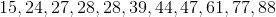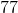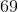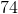Explanation:

To find the range of a set subtract the smallest number in the set from the largest number in the set: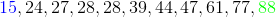The largest number is in green: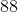The smallest number is in blue:Therefore the range is,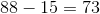.

### Example Question #81 : Basic Statistics

Find the range of the following set of data.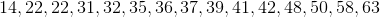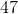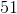Explanation:

To find the range of a set subtract the smallest number in the set from the largest number in the set: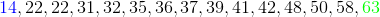The largest number is in green:The smallest number is in blue:Therefore the range is their difference,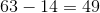.

### All Algebra II Resources